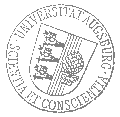# Universität AugsburgInstitut für MathematikOberseminar zur Algebra und Zahlentheorie

Claude Sabbah
École polytechnique

spricht am

Donnerstag, 4. Mai 2023

um

12:30 Uhr

im

Raum 2004 (L1)

über das Thema:

## »Irregular Hodge theory, periods, arithmetic«

 Abstract: This talk aims at illustrating, with the arithmetic example of the functional equation for certain exponential sums (Kloosterman moments), various properties of mathematical research: The interest of mixing mathematical techniques from various domains. The time constant that can be long between the emergence of a mathematical idea and its full realization. The incredible ability of physicists to understand mathematical theories and to provide mathematicians with challenging conjectures. This is a joint work with Javier Fresán and Jeng-Daw Yu.

 Hierzu ergeht herzliche Einladung. Prof. Dr. Marco Hien

[Impressum]      [Datenschutz]      wwwadm@math.uni-augsburg.de,     Mi 12-Apr-2023 11:31:20 MESZ